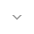``` public class UserSensorReading ```
``` extends Object ``` ``` ```

Readings consist of an array of floats as well as a sensor status to indicate the confidence level of the given values. The meaning and order of the float array depends on the sensor type, and must match the conventions described by `android.hardware.SensorEvent.values`.

Sensor status is not used for all sensor types; those which do not will ignore the reported status.

## Summary

### Public constructors

``` UserSensorReading(float[] values) ```

Creates a new UserSensorReading with a default `SENSOR_STATUS_ACCURACY_HIGH` status.

``` UserSensorReading(float[] values, int status) ```

### Public methods

``` boolean``` ``` equals(Object object) ```

Indicates whether some other object is "equal to" this one.

``` int``` ``` hashCode() ```

Returns a hash code value for the object.

### Inherited methodsFrom class ``` java.lang.Object ```

## Public constructors

`UserSensorReading (float[] values)`

Creates a new UserSensorReading with a default `SENSOR_STATUS_ACCURACY_HIGH` status.

`values` are copied here so may be safely re-used by the caller.

Parameters
`values` `float`: sensor reading data

```UserSensorReading (float[] values,
int status)```

`values` are copied here so may be safely re-used by the caller.

Parameters
`values` `float`: sensor reading data

`status` `int`: `android.hardware.SensorManager.SENSOR_STATUS_*` value

## Public methods

### equals

`boolean equals (Object object)`

Indicates whether some other object is "equal to" this one.

The `equals` method implements an equivalence relation on non-null object references:

• It is reflexive: for any non-null reference value `x`, `x.equals(x)` should return `true`.
• It is symmetric: for any non-null reference values `x` and `y`, `x.equals(y)` should return `true` if and only if `y.equals(x)` returns `true`.
• It is transitive: for any non-null reference values `x`, `y`, and `z`, if `x.equals(y)` returns `true` and `y.equals(z)` returns `true`, then `x.equals(z)` should return `true`.
• It is consistent: for any non-null reference values `x` and `y`, multiple invocations of `x.equals(y)` consistently return `true` or consistently return `false`, provided no information used in `equals` comparisons on the objects is modified.
• For any non-null reference value `x`, `x.equals(null)` should return `false`.

The `equals` method for class `Object` implements the most discriminating possible equivalence relation on objects; that is, for any non-null reference values `x` and `y`, this method returns `true` if and only if `x` and `y` refer to the same object (`x == y` has the value `true`).

Note that it is generally necessary to override the `hashCode` method whenever this method is overridden, so as to maintain the general contract for the `hashCode` method, which states that equal objects must have equal hash codes.

Parameters
`object` `Object`: the reference object with which to compare.

Returns
`boolean` `true` if this object is the same as the obj argument; `false` otherwise.

### hashCode

`int hashCode ()`

Returns a hash code value for the object. This method is supported for the benefit of hash tables such as those provided by `HashMap`.

The general contract of `hashCode` is:

• Whenever it is invoked on the same object more than once during an execution of a Java application, the `hashCode` method must consistently return the same integer, provided no information used in `equals` comparisons on the object is modified. This integer need not remain consistent from one execution of an application to another execution of the same application.
• If two objects are equal according to the `equals(Object)` method, then calling the `hashCode` method on each of the two objects must produce the same integer result.
• It is not required that if two objects are unequal according to the `equals(java.lang.Object)` method, then calling the `hashCode` method on each of the two objects must produce distinct integer results. However, the programmer should be aware that producing distinct integer results for unequal objects may improve the performance of hash tables.

As much as is reasonably practical, the hashCode method defined by class `Object` does return distinct integers for distinct objects. (This is typically implemented by converting the internal address of the object into an integer, but this implementation technique is not required by the Java™ programming language.)

Returns
`int` a hash code value for this object.

[]
[]